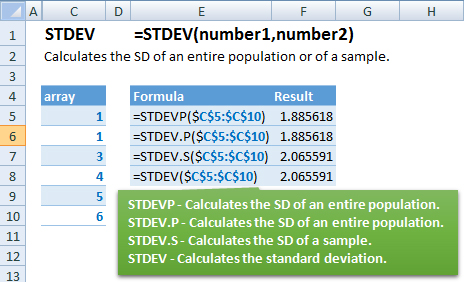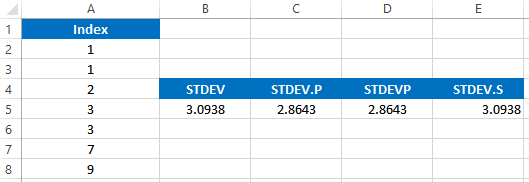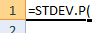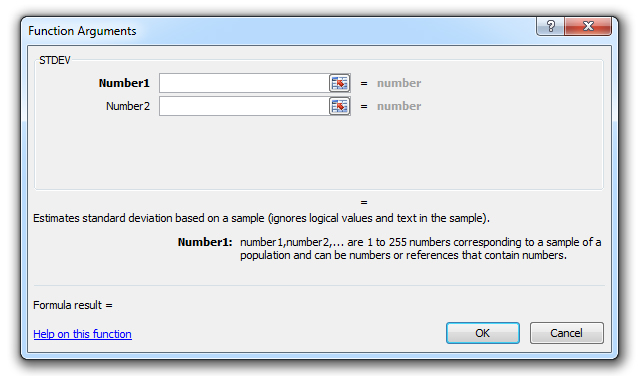### STDEV Formula – Standard Deviation – Excel, VBA, & G Sheets

This Excel Tutorial demonstrates how to use the Excel STDEV Function in Excel to calculate the standard deviation, with formula examples.

## STDEV Function Description:

The STDEV Function Calculates the standard deviation. Ignores text, logical valus, and blank cells.

## Formula Examples:AutoMacro - VBA Code Generator

## Syntax and Arguments:

The Syntax for the STDEV Formula is:

`=STDEV(number1,number2)`

### Function Arguments ( Inputs ):

array – An array of numbers.

## STDEV Examples in VBA

You can also use the STDEV function in VBA. Type:
`application.worksheetfunction.stdev(number1,number2)`

Executing the following VBA statements

```Range("B5") = Application.WorksheetFunction.StDev(Range("A2:A8"))
Range("C5") = Application.WorksheetFunction.StDev_P(Range("A2:A8"))
Range("D5") = Application.WorksheetFunction.StDevP(Range("A2:A8"))
Range("E5") = Application.WorksheetFunction.StDev_S(Range("A2:A8"))
```

will produce the following resultsFor the function arguments (array, etc.), you can either enter them directly into the function, or define variables to use instead.

Return to the List of all Functions in Excel

## How to use the STDEV Function in Excel:

To use the AND Excel Worksheet Function, type the following into a cell:
`=AND(`
After entering it in the cell, notice how the AND formula inputs appear below the cell:You will need to enter these inputs into the function. The function inputs are covered in more detail in the next section. However, if you ever need more help with the function, after typing “=STDEV(” into a cell, without leaving the cell, use the shortcut CTRL + A (A for Arguments) to open the “Insert Function Dialog Box” for detailed instructions:For more information about the STDEV Formula visit the
Microsoft Website.

## Excel Practice Worksheet

Practice Excel functions and formulas with our 100% free practice worksheets!

• Automatically Graded Exercises
• Learn Excel, Inside Excel!